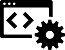#開発ツール

## SMOOSY(Ito, K. et al. Commun. Chem.,2020)

Spatial MOlecular-dynamically Ordered NMR SpectroscopY (SMOOSY) of intact bodies and heterogeneous systems

## Prediction of carbonless NMR spectra by the machine learning of theoretical and fragment descriptors(Ito, K. et al. Anal.Chem., 2021)

Improved prediction of carbonless NMR spectra by the machine learning of theoretical and fragment descriptors for environmental mixture analysis.

## Exploratory machine-learned theoretical chemical shifts(Ito, K. et al. Chem. Sci., 2018)

The chemical shift predictive tool that combines quantum chemistry and machine learning.

## Fragment Assembly Approach(Ito, K. et al. ACS Chem. Biol., 2016)

Fragment assembly approach based on graph/network theory with quantum chemistry verifications for assigning multidimensional NMR signals in metabolite mixtures

## Solubility Prediction(Kurotani, A. et al. ACS Omega, 2021)

A solubility prediction webtool using a unique machine learning method called the in-phase deep neural network (ip-DNN), which starts exclusively from the analytical input data (e.g., NMR information, refractive index, and density) to predict solubility by predicting intermediate elements, such as molecular components and molecular descriptors, in the multiple-step method.

## Decomposition Factor Analysis(Yamawaki, R. et al. Appl. Sci., 2021)

Decomposition Factor Analysis Based on Virtual Experiments throughout Bayesian Optimization for Compost-Degradable Polymers

## Signal Deconvolution and GTMR for Solid-State NMR of Multi-Component Materials(Yamada, S. et al. Int. J. Mol. Sci., 2021)

Python tools based on signal deconvolution methods using a short-time Fourier transform (STFT) and a non-negative tensor/matrix factorization (NTF, NMF), and methods for predicting NMR signals and physical properties using generative topographic mapping regression (GTMR) for solid-state NMR analysis of multi-component materials.

## Signal Deconvolution and Noise Factor Analysis(Yamada, S. et al. Int. J. Mol. Sci., 2020)

NMR measurement informatics tool for data cleansing that combines short-time Fourier transform (STFT; a time–frequency analytical method) and probabilistic sparse matrix factorization (PSMF) for signal deconvolution and noise factor analysis

## InterSpin(Yamada, S. et al. ACS Omega, 2019)

Integrated supportive webtools for low- and high-field NMR analysis toward molecular complexities

## Ecomics(Ogata, Y. et al. PLoS One, 2012)

Web tools for environmental and metabolic systems

## An integrated strategy linking three analytical (machine learning, factor mapping, and forecast-error-variance decomposition) approaches(Oita, A. et al. Sci. Total Environ., 2018)

Analytical tool for profiling physicochemical and planktonic features from discretely/continuously sampled surface water

## Ensemble deep neural network(Asakura, T. et al. Anal. Chimica Acta, 2018)

The ensemble deep neural network (EDNN) regression for fish size based on metabolic profiles was superior to that of DNN, random forest, and support vector machine algorithms.

## Deep neural network-mean decrease accuracy(Date, Y. & Kikuchi, J. Anal. Chem., 2018)

An improved DNN-based analytical approach that incorporates an importance estimation for each variable using a mean decrease accuracy (MDA) calculation, which is based on a permutation algorithm; this approach is called DNN-MDA.

## Kernel principal component analysis and computational machine learning(Shiokawa, Y. et al. Sci. Rep., 2018)

Kernel principal component analysis (KPCA), random forest and market basket analysis -incorporated analytical approach for extracting useful information from metabolic profiling data.